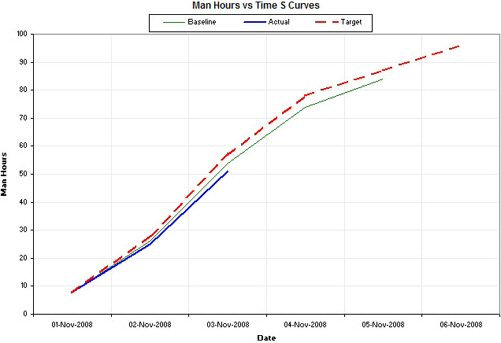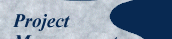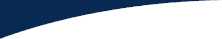This paper was originally authored by Greg Gendall of Midori Media and published by Project Magazine in May 2003. It has since been revised and updated by the present author. It was submitted for publication by Email, February 16, 2009, and is copyright © David Garland. Published here May 2009.

## Actual versus Target

### Target Man Hours versus Time S-curve

To generate the Actual and Target S-curves, a Production Schedule is required. A Production Schedule for this project is shown below.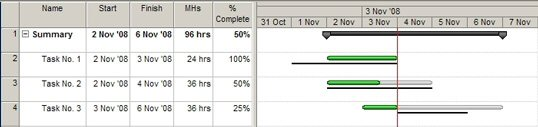##### Figure 13: Production Schedule

To generate a Target Man Hours versus Time S-curve, two sets of calculations are necessary. The first set of calculations is performed for each task in the Production Schedule.

1. Calculate the duration in days for each task
i.e. Duration = Finish Date - Start Date + 1
2. Calculate Man Hours per day for each task
i.e. MHs per Day = Man Hours / Duration

These calculations are shown in the following table.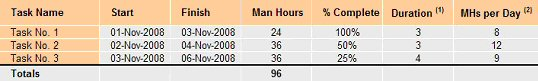##### Figure 14: Target S-curve Calculation 1 of 2

The second set of calculations is performed for each day in the Production Schedule.

1. Calculate the total Man Hours per Day for all tasks.
2. Calculate the Year To Date Total for Man Hours per Day for all tasks.

These calculations are shown in the following table: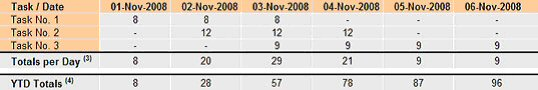##### Figure 15: Target S-curve Calculation 2 of 2

The resulting Target Man Hours versus Time S-curve is shown below.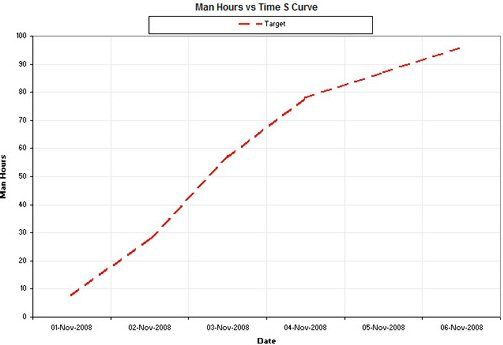### Actual Man Hours versus Time S-curve

To generate an Actual Man Hours versus Time S-curve, two sets of calculations are performed, but before these calculations may be performed, the Cut Off Date needs to be defined. This is the date the Production Schedule was last updated. For this example a Cut Off Date of 3rd November 2008 will be used.

The first set of calculations is performed for each task in the Production Schedule.

1. Determine the Task Status: Complete, Ongoing, Not Started.
Complete: Tasks that have a Finish Date prior to the Cut Off Date.
Ongoing: Tasks that have commenced and have a Finish Date on or after the Cut Off Date.
Not Started: Tasks that have a Start Date after the Cut Off Date.
2. Calculate the Duration to Date based on the Task Status.
Complete: Duration To Date = Finish Date - Start Date + 1
Ongoing: Duration To Date = Cut Off Date - Start Date + 1
Not Started: Duration To Date = 0.
3. Calculate Man Hours to Date for Complete and Ongoing Tasks.
i.e. Man Hours to Date = Man Hours x % Complete / Duration to Date.

These calculations are shown in the following table.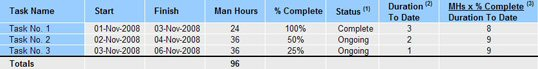##### Figure 17: Actual S-curve Calculation 1 of 2

The second set of calculations is performed for each day in the Production Schedule up to the Cut Off Date.

1. Calculate the total Man Hours to Date per Day for all tasks.
2. Calculate the Year To Date Total for Man Hours to Date per Day for all tasks.

These calculations are shown in the following table.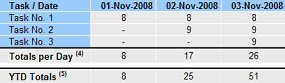##### Figure 18: Actual S-curve Calculation 2 of 2

The resulting Actual Man Hours versus Time S-curve is shown below.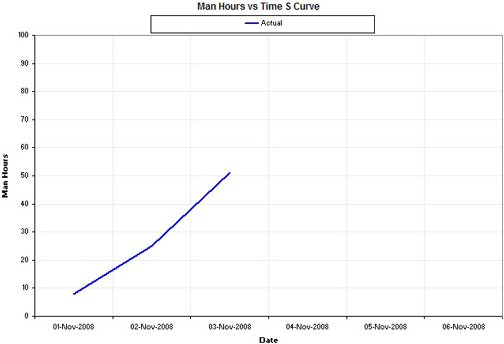##### Figure 19: Actual Man Hours versus Time S-curve

The Baseline, Actual, and Target S-curves are usually combined, as shown below.